# Different Types of Curves - Closed Curve, Open Curve, Simple Curve.

#### definition

Closed Curves: Figures in which initial and endpoints coincide with each other are called closed figures.

Region: The interior of a curve together with its boundary is called its region.

Open Curves: A curve has two endpoints, and when it does not enclose the area within itself it is known as an open curve.

Simple Curves: Simple curve are those curves which changes direction but does not cross itself while changing direction.

Non-simple curve: If a curve does cross itself, then it is called a Non-simple curve.

# Different Types of Curves:

### 1. Closed Curve:

• Figures in which initial and endpoints coincide with each other are called closed figures.

• A closed curve is formed by joining the endpoints of an open curve together.

• A closed curve has no endpoints and encloses an area (or a region).
Examples, Circles, ellipses are form of closed curves.

•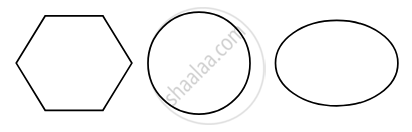• In a closed curve, there are three parts.
(i) interior (‘inside’) of the curve
(ii) boundary (‘on’) of the curve and
(iii) exterior (‘outside’) of the curve.

•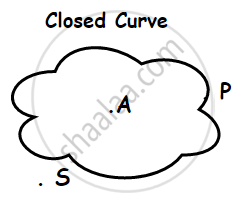• The interior of a curve together with its boundary is called its “region”.

### 2. Open Curve:

• A curve has two endpoints, and when it does not enclose the area within itself it is known as an open curve.

• An open curve does not enclose an area within itself and it has two endpoints.

•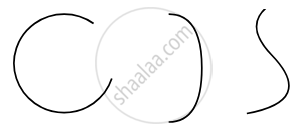### 3. Simple Curve:

• A curve changes its direction but does not cross itself while changing direction. This type of curve is known as a simple curve.

• A simple curve may be open or closed.

• If a curve does cross itself, then it is called a Non-simple curve.

•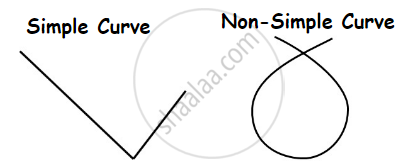### Simple closed curve: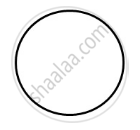### Simple open curve: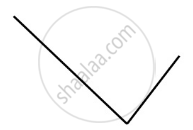### A closed curve that is not simple: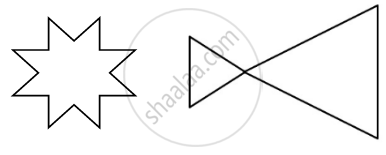An Open curve that is not simple: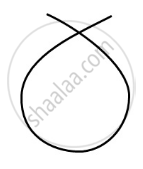If you would like to contribute notes or other learning material, please submit them using the button below.

### Shaalaa.com

What Are Open And Closed Curves? [00:01:55]
S
0%